# 2021年银行职业资格考试必背公式

(1)单期中的终值：

FV=C0(1+r)

(2)单期中的现值：

PV=C1(1+r)

(3)多期的终值和现值：

FV=PV×(1+r)t

PV=FV(1+r)t

(1)复利期间

FV=C0×1+rmmt

(2)有效年利率(EAR)

EAR=1+rmm-1

(1)年金现值的公式为：PV=Cr1-1(1+r)t。

(2)期初年金现值的公式为：PV期初=Cr1-1(1+r)t(1+r)。

(3)年金终值的公式为：FV=Cr×[(1+r)t-1]。

(4)期初年金终值的公式为：PV期初=Cr×[(1+r)t-1](1+r)。

E(Ri)=[P1R1+P2R2+…+PnRn]×100%=∑PiRi×100%

“意外”计算

2021银行职业资格考试辅导课程，视频课程+考试题库！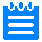学习计划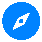考试指南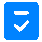高频考点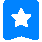历年真题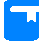精品课程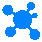思维导图